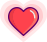# Rust 能取代 Python，更好的实现神经网络？Rust 也能实现神经网络？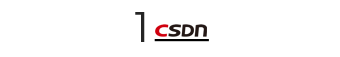Python的实现




class Network(object):

def __init__(self, sizes):
"""The list sizes contains the number of neurons in the
respective layers of the network.  For example, if the list
was [2, 3, 1] then it would be a three-layer network, with the
first layer containing 2 neurons, the second layer 3 neurons,
and the third layer 1 neuron.  The biases and weights for the
network are initialized randomly, using a Gaussian
distribution with mean 0, and variance 1.  Note that the first
layer is assumed to be an input layer, and by convention we
won't set any biases for those neurons, since biases are only
ever used in computing the outputs from later layers."""
self.num_layers = len(sizes)
self.sizes = sizes
self.biases = [np.random.randn(y, 1) for y in sizes[1:]]
self.weights = [np.random.randn(y, x)
for x, y in zip(sizes[:-1], sizes[1:])]




def update_mini_batch(self, mini_batch, eta):
"""Update the network's weights and biases by applying
gradient descent using backpropagation to a single mini batch.
The mini_batch is a list of tuples (x, y), and eta
is the learning rate."""
nabla_b = [np.zeros(b.shape) for b in self.biases]
nabla_w = [np.zeros(w.shape) for w in self.weights]
for x, y in mini_batch:
delta_nabla_b, delta_nabla_w = self.backprop(x, y)
nabla_b = [nb+dnb for nb, dnb in zip(nabla_b, delta_nabla_b)]
nabla_w = [nw+dnw for nw, dnw in zip(nabla_w, delta_nabla_w)]
self.weights = [w-(eta/len(mini_batch))*nw
for w, nw in zip(self.weights, nabla_w)]
self.biases = [b-(eta/len(mini_batch))*nb
for b, nb in zip(self.biases, nabla_b)]

backprop函数在计算该神经网络的代价函数的梯度时，首先从输入图像的正确输出开始，然后将错误反向传播至网络的各层。这需要大量的数据调整，在将代码移植到Rust时我在此花费了大量的时间，在此篇幅有限，我无法深入讲解，如果你想了解具体的详情，请参照这本书（http://neuralnetworksanddeeplearning.com/chap2.html）。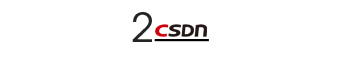Rust的实现




use ndarray::Array2;

#[derive(Debug)]
struct Network {
num_layers: usize,
sizes: Vec<usize>,
biases: Vec<Array2<f64>>,
weights: Vec<Array2<f64>>,
}




use rand::distributions::StandardNormal;
use ndarray::{Array, Array2};
use ndarray_rand::RandomExt;

impl Network {
fn new(sizes: &[usize]) -> Network {
let num_layers = sizes.len();
let mut biases: Vec<Array2<f64>> = Vec::new();
let mut weights: Vec<Array2<f64>> = Vec::new();
for i in 1..num_layers {
biases.push(Array::random((sizes[i], 1), StandardNormal));
weights.push(Array::random((sizes[i], sizes[i - 1]), StandardNormal));
}
Network {
num_layers: num_layers,
sizes: sizes.to_owned(),
biases: biases,
weights: weights,
}
}
}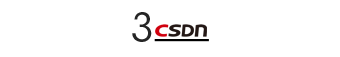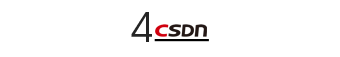impl Network {
fn update_mini_batch(
&mut self,
training_data: &[MnistImage],
mini_batch_indices: &[usize],
eta: f64,
) {
let mut nabla_b: Vec<Array2<f64>> = zero_vec_like(&self.biases);
let mut nabla_w: Vec<Array2<f64>> = zero_vec_like(&self.weights);
for i in mini_batch_indices {
let (delta_nabla_b, delta_nabla_w) = self.backprop(&training_data[*i]);
for (nb, dnb) in nabla_b.iter_mut().zip(delta_nabla_b.iter()) {
*nb += dnb;
}
for (nw, dnw) in nabla_w.iter_mut().zip(delta_nabla_w.iter()) {
*nw += dnw;
}
}
let nbatch = mini_batch_indices.len() as f64;
for (w, nw) in self.weights.iter_mut().zip(nabla_w.iter()) {
*w -= &nw.mapv(|x| x * eta / nbatch);
}
for (b, nb) in self.biases.iter_mut().zip(nabla_b.iter()) {
*b -= &nb.mapv(|x| x * eta / nbatch);
}
}
}




fn to_tuple(inp: &[usize]) -> (usize, usize) {
match inp {
[a, b] => (*a, *b),
_ => panic!(),
}
}

fn zero_vec_like(inp: &[Array2<f64>]) -> Vec<Array2<f64>> {
inp.iter()
.map(|x| Array2::zeros(to_tuple(x.shape())))
.collect()
}




let (delta_nabla_b, delta_nabla_w) = self.backprop(&training_data[*i]);




for (nb, dnb) in nabla_b.iter_mut().zip(delta_nabla_b.iter()) {
*nb += dnb;
}
for (nw, dnw) in nabla_w.iter_mut().zip(delta_nabla_w.iter()) {
*nw += dnw;
}




let nbatch = mini_batch_indices.len() as f64;
for (w, nw) in self.weights.iter_mut().zip(nabla_w.iter()) {
*w -= &nw.mapv(|x| x * eta / nbatch);
}
for (b, nb) in self.biases.iter_mut().zip(nabla_b.iter()) {
*b -= &nb.mapv(|x| x * eta / nbatch);
}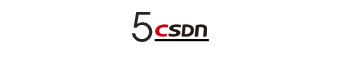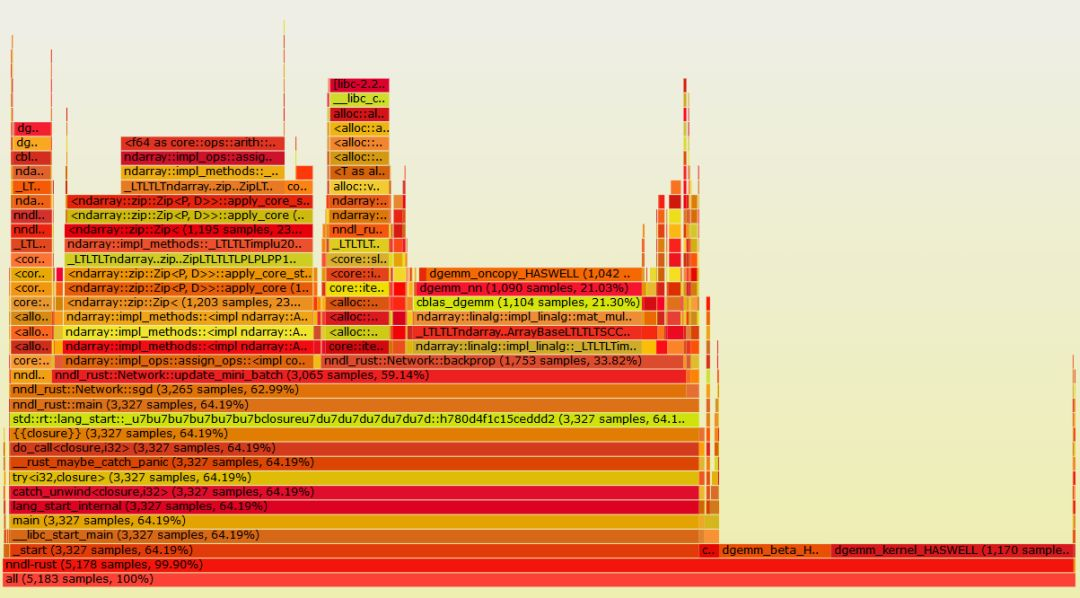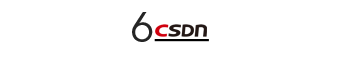Rust是否适合数据科学工作流程？

【End】

https://edu.csdn.net/topic/python115?utm_source=csdn_bw热 文 推 荐

☞这位博士都 50 多岁了，为啥还在敲代码？

☞C# 导出 Excel 的 6 种简单方法！你会几种？

☞这位博士都 50 多岁了，为啥还在敲代码？

☞给面试官讲明白：一致性Hash的原理和实践

☞她说：行！没事别嫁程序员！点击阅读原文，输入关键词，即可搜索您想要的 CSDN 文章。


`